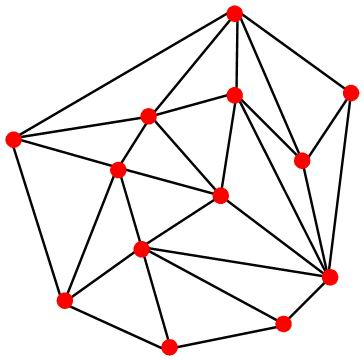Online JudgeProblem SetAuthorsOnline ContestsUser
Web Board
F.A.Qs
Statistical Charts
Problems
Submit Problem
Online Status
Prob.ID:
Register
Authors ranklist
Current Contest
Past Contests
Scheduled Contests
Award Contest
Register
Language:
Triangularize the Convex Hull
 Time Limit: 2000MS Memory Limit: 65536K Total Submissions: 401 Accepted: 68

Description

Given N points (we call them initial points) in a plane, there may be several ways to triangularize the convex hull of these points. Here the convex hull of a set Q of points is the smallest convex polygon P for which each point in Q is either on the boundary of P or in its interior. To triangularize the convex hull means to split the convex hull into several triangles. The triangles should meet the following request:
1. The vertices of the triangles are the N initial points.
2. On an edge of a triangle (not including the tops of the edge), there won't be any initial points.
3. No two triangles overlap with each other, i.e. the area of all the triangles is equal to the area of the convex hull.

The following figure is a example of triangularizing the convex hull of the initial points. It's easy to know that there may be many different ways to triangularize the convex hull and number of triangles, which are split out by an arbitrary triangulation, is fixed. If a triangulation split the convex hull into M triangles, we may get 3*M angles (because every triangle contains 3 angles). Your job is to find a triangulation such that the minimum angle among the 3*M angles of the triangulation is not smaller than the minimum angle of any triangulation else.You may assume that all the points cannot be in a straight line, and no two points can be at the same position.

Input

The input contains several test cases. In each case, the first line contains an integer N (3<= N <= 100). Then come N line, in each line there are two integers Xi and Yi (0 <= Xi, Yi < 100), which is the coordinates of an initial point.

Output

For each case, output a float number, which is rounded to two digits after the decimal point, in a single line. The float number is the size of minimum angle of the triangulation that you are ask to get.

Sample Input

```9
0 0
0 1
0 2
1 0
1 1
1 2
2 0
2 1
2 2
3
1 1
1 0
0 1
4
0 0
5 1
0 1
1 2
```

Sample Output

```45.00
45.00
18.43
```

Source

[Submit]   [Go Back]   [Status]   [Discuss]Home PageGo BackTo top